# r-一致D-超图的最大边数The Maximum Number of Hyperedges of An r-Uniform D-Hypergraph

• 全文下载: PDF(339KB)    PP.105-108   DOI: 10.12677/AAM.2020.91013
• 下载量: 65  浏览量: 95

A mixed hypergraph on a finite set X is a triple H=（X,C,D）, where C and D are families of subset of X. The member of C is called C-edge and the member of D is called D-edge. A mixed hypergraph is called C-hypergraph when D=∅, a mixed hypergraph is called D-hypergraph when C=∅. Let H=（X,C,D） be a mixed hypergraph, r is a positive integer not less than 2. For an arbitrary C-edge and D-edge, if we have |C|=r|D|=r, then the mixed hypergraph H is called r-uniform mixed hypergraph. In particular, if , the mixed hypergraph H is called r-uniform mixed D-hypergraph. In this paper, we solve the problem about the maximum number of hyperedges of an r-uniform D-hypergraph when χ（H）=k.

1. 引言

1995年，Vitaly Voloshin  对于超图的染色理论提出了其对偶问题，即：使某些超边中至少有两个点染相同的颜色，此类超边称为C-边，传统超边称为D-边，同时包含C-边和D-边的超图称为混合超图，记为 $H=\left(X,C,D\right)$ 。这两种超图的主要区别体现在染色上。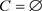的混合超图称为D-超图，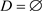的混合超图称为C-超图。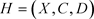是一混合超图，H的一个正常的k-染色是一个映射 $\phi$$X\to \left\{1,2,\cdots ,k\right\}$ ，使得下述条件成立：

1) 每条C-边中至少有两个顶点染相同的颜色；

2) 每条D-边中至少有两个顶点染不同的颜色。

$H=\left(X,C,D\right)$ 是一混合超图，r是不小于2的正整数，若满足对任意的C-超边和D-超边，都有 $|C|=r$$|D|=r$ ，则称混合超图H为r-一致混合超图。特别地，若又有 $C=\varnothing$ ()，则称H为r-一致D-超图(r-一致C-超图)。

2. 定理及其证明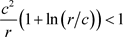$H=\left(X,D\right)$ 具有 $\epsilon \left(H\right)={k}^{r-1}c$ 条边且p满足上述条件。首先我们随机地用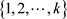中的一种颜色逐个给H的顶点着色。其次对于每个顶点v，我们抛掷硬币，出现正面的概率为p。另外，对V中的顶点随机排序。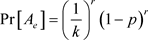$k\underset{e\in H}{\sum }\mathrm{Pr}\left[{A}_{e}\right]=c{\left(1-p\right)}^{r}$

1) 边 $e,f$ 恰重叠一个元素，记作v；

2) 在第一次染色中边f是单色的且在最终的染色中e是单色的；

3) 在步骤2中，点v是边e中最后一个改变颜色的点；

4) 当考虑点v时，边f仍然是单色的；

$\mathrm{Pr}\left[{C}_{ef}|\sigma \right]\le {\left(\frac{1}{k}\right)}^{r}p{\left(1-p\right)}^{j}{\left(\frac{1}{k}\right)}^{r-i-1}{\left(\frac{1+p}{k}\right)}^{i}$

$\mathrm{Pr}\left[{C}_{ef}\right]\le {k}^{1-2r}pE\left[{\left(1+p\right)}^{i}{\left(1-p\right)}^{j}\right]$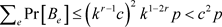Voloshin, V.I. (2002) Coloring Mixed Hypergraphs: Theory, Algorithms and Applications, AMS, Providence.  Bujt´as, C. and Tuza, Z. (2008) Uniform Mixed Hypergraphs: The Possible Numbers of Colors. Graphs and Combinatorics, 24, 1-12. https://doi.org/10.1007/s00373-007-0765-5  Diao, K., Zhao, P. and Wang, K. (2014) The Smallest One-Realization of a Given Set III. Graphs and Combinatorics, 30, 875-885. https://doi.org/10.1007/s00373-013-1322-z  Voloshin, V.I. (1992) On the Upper Chromatic Number of a Hypergraph. Scientific Research Conference of the Moldova State University, Theses of Resports, Kishinev, Vol. 1, 42.  Cai, J., Xiong, Y. and Yang, D. (2020) A Note on the Maximum Number of Hyperedge so f C-Hypergraph. Submitted for Publication.  Alon, N. and Spencer, J.H. (2008) The Probablistic Method. 3rd Edition, John Wiley and Sons, New York.